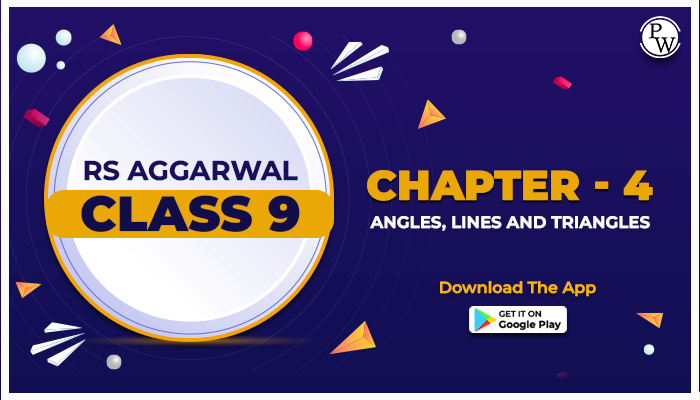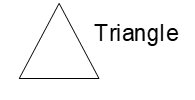# RS Aggarwal Class 9 Chapter - 4 Angles, Lines and Triangles

## Line

A line is a one-dimensional figure, long but not wide. The line comprises a set of points extending in the opposite direction. Two points are determined on a two-dimensional plane. Two points lie on the same side are called collinear points. or

A line is known as a straight set of points that extend across opposite sides

• Infinite in both directions (infinite)
• It has no thickness
• it has one side### Types of Line

There are four types of lines:

• Horizontal Lines
• Vertical Lines
• Parallel Lines
• Perpendicular Lines

### Angle

An angle is a geometrical shape formed by combining two rays at its ends. An angle can also be represented by three shapes that define an angle, the letter in the middle being where the angle exists (i.e., its vertex). Angles are usually represented by Greek letters such as θ, α, β, etc.e.g. ∠ABC, where B is the given angle.

### Types of Angles

• Acute Angle: It lies between 0° to 90.
• Obtuse Angle: It lies between 90° to 180°
• Right Angle: The angle which is exactly equal to 90°
• Straight Angle: The angle which is exactly equal to 180°
• Reflex Angle: The angle which is greater than 180 degrees and less than 360 degrees
• Full Rotation: The complete rotation of angle equal to 360 degrees

### Triangle

In Geometry, a triangle is a three-sided polygon consisting of three edges and three vertices. The most important property of a triangle is that the sum of the inner angles of the triangle is equal to 180 degrees. This property is called the angle sum property of a triangle. Sum of angle of triangle is 1800.

If ABC is a triangle, it is denoted as ∆ABC, where A, B, and C are triangles. In Euclidean geometry, a triangle is a two-dimensional shape that appears as three non-collinear points in a unique plane.

### Types of Triangle

On the basis of length of the sides

• Scalene Triangle
• Isosceles Triangle
• Equilateral Triangle

On the basis of measurement of the angles

• Acute Angle Triangle
• Right Angle Triangle
• Obtuse Angle Triangle

Academic team of Physics Wallah prepared a step by step RS aggarwal class 9 solutions of chapter-4 Angles, Lines and Triangles. RS Aggarwal class 9 solution for chapter-4 Angles, Lines and Triangles Exercise-4C is uploaded for reference only don’t copy the solutions.

Read the theory Given in NCERT text book and solve all numericals given in the exercise use Physics Wallah NCERT solutions for class 9 Maths. For additional information related to the subject you can check the Maths Formula section.

The below mentioned PDF is RS Aggarwal Class 9 solutions for Chapter- 4 Angles, Lines and Triangles Exercise - 4C